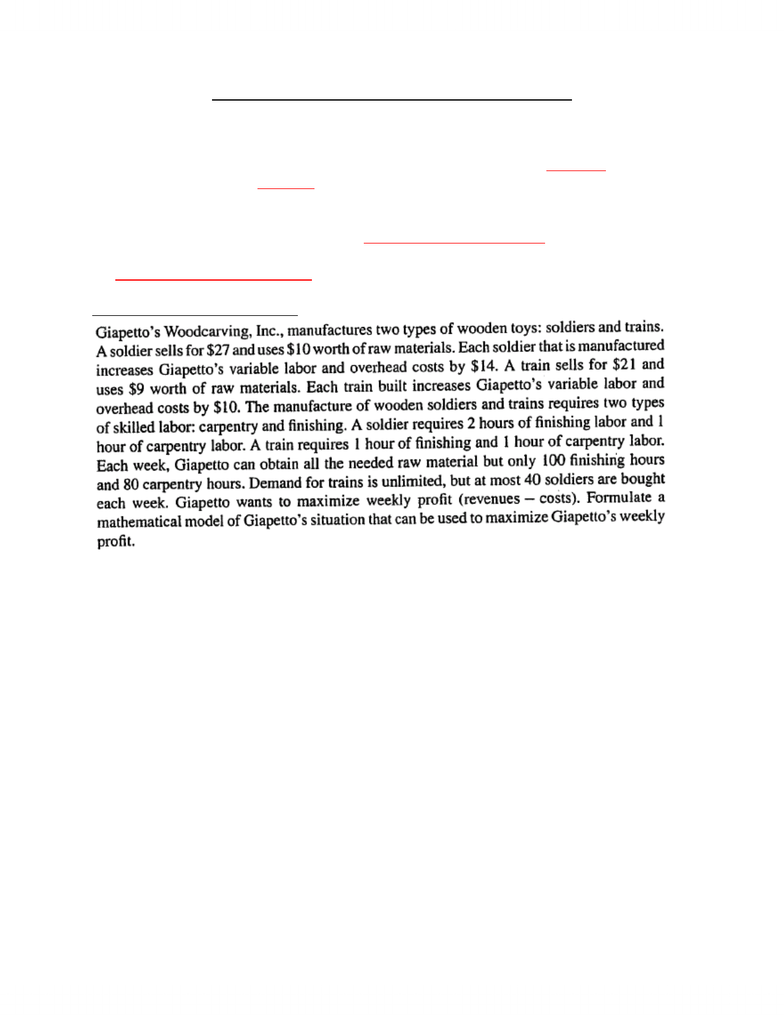# MGOC10H3 Lecture Notes - Nonlinear Programming, Integer Programming, Production Planning

24 views3 pages
School
Course
ProfessorMGTC74 Analysis for Decision Making
Lecture 01
Chapter 2 – LP Model Formulation & Solution
Basic components of linear programming (LP) model:
1) Define decision variables—variables that describes the decisions to be made
2) State objective function—in LP model decision maker will want to maximize (e.g., profit,
output, revenue) or minimize (cost, risk) some function of decision variables
3) Specify constraints
4) Sign restrictions or variables—in general we can have x≥0, x≤0, x unrestricted in sign
if x1 ,x2 must be integer, then we have an integer programming problem
if either objective function or any of the constraints is nonlinear e.g., 3x12 + 2x2 then we have
nonlinear programming problem
Example – Production Planning
1) x1 = # of soldiers to make each week
x2 = # of trains to make each week
2) objective is to maximize profits
Profit = (27x1 + 21x2) – (10x1 + 9x2+14x1 + 10x2) = 3x1 + 2x2
3) constraint 1 no more than 100 hrs finishing time used
finish time soldier + finish time trains ≤ 100hr 2x1 + 1x2 ≤100
constraint 2 no more than 80hrs carpentry time used
1x1 + 1x2 ≤80
constraint 3 at most 40 soldiers can be sold
x1 < 40
4) cannot produce negative # of soldiers/trains so x1,x2 > 0
Final LP model
Max z = 3x1 + 2x2
2x1 + 1x2 ≤100 (finishing)
1x1 + 1x2 ≤ 0 (carpentry)
x1 < 40 (soldier demand)
x1, x2 > 0
1
Unlock document

This preview shows page 1 of the document.
Unlock all 3 pages and 3 million more documents.

## Document Summary

Chapter 2 lp model formulation & solution. Example production planning: x1 = # of soldiers to make each week x2 = # of trains to make each week, objective is to maximize profits. 1x1 + 1x2 80 constraint 3 at most 40 soldiers can be sold x1 < 40: cannot produce negative # of soldiers/trains so x1,x2 > 0. 1x1 + 1x2 0 x1 < 40 x1, x2 > 0 (finishing) (carpentry) (soldier demand) For every gallon a produced at least 3 gallons of b must be produced. A, b 0 (production regular) (custom order) (processing) (production required) Z = 50000c + 100000f z = 50c + 100f. C, f > 0 (at least 28 million w) (at least 24 million m) Max z = 0. 06b + 0. 1s + 0. 02m. B 0. 3 or b 0. 3 (b + s + m)